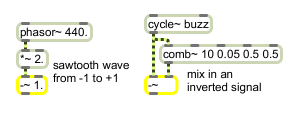# -~

Signal subtraction

## Description

-~ is a signal minus-operator that outputs a signal which is the difference between two signals.

## Arguments

Name Type Opt Description
initial-subtraction-value float or int opt Sets an initial amount to subtract from the signal coming into the left inlet. If a signal is connected to the right inlet, the argument is ignored. If no argument is present, and no signal is connected to the right inlet, the initial value is 0 by default.

## Messages

 int input [int] In left inlet: Subtracts the signal coming into the right inlet from this value. If a signal is also connected to the left inlet, a float or int is ignored. In right inlet: An amount to subtract from the signal coming into the left inlet. If a signal is also connected to the right inlet, a float or int is ignored. float input [float] In left inlet: Subtracts the signal coming into the right inlet from this value. If a signal is also connected to the left inlet, a float or int is ignored. In right inlet: An amount to subtract from the signal coming into the left inlet. If a signal is also connected to the right inlet, a float or int is ignored. signal In left inlet: The signal coming into the right inlet or a constant value received in the right inlet is subtracted from this signal. In right inlet: The signal is subtracted from the signal coming into the left inlet, or a constant value received in the left inlet.

## Output

signal: The difference between the two inputs.

## ExamplesNegative DC offset... Subtraction used to invert a signal before adding it in